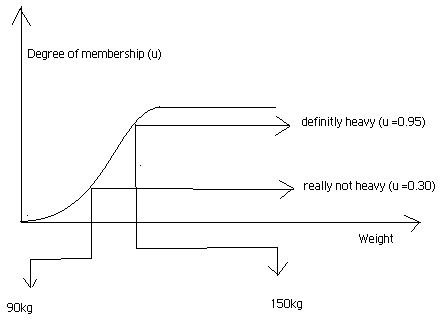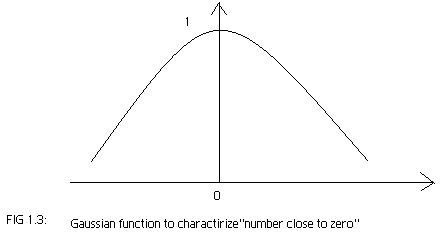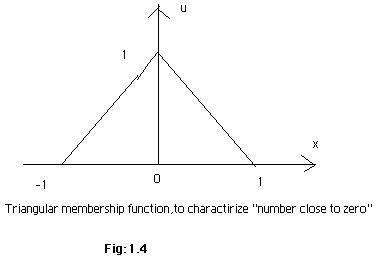# Fuzzy logic implementation - Membership functions explained

Page content

## Fuzzy logic implementation - What are membership functions?

Definition: A graph that defines how each point in the input space is mapped to membership value between 0 and 1. Input space is often referred as the universe of discourse or universal set (u), which contain all the possible elements of concern in each particular application.

Explanation: Suppose we have a set of heavy weight people ranging 80-200 kg in weight. In this case the universe of discourse is the weight (80-200kg).We might say that people heavier then 80kg are heavy. But such distinction is clearly absurd, as it is not clear that by what degree one is heavier then other. The right way of defining the set of heavy people will be the graphical representation, showing a smooth varying cure that passes from not heavy to heavy. Fig (a) and (b) shows a graphical representation. The output axis is a number known as membership value, between 0 and 1.The transition from non heavy to heavy is known as membership function, and is often given designation (μ). Both people can heavier to same degree, but one significantly heavy then other

Types of membership functions

Before we start defining different types of membership functions, let us consider a fuzzy IF-THEN rule for a car:

• IF the speed of a car is high, THEN apply less force to the accelerator (1.1)

• IF the speed is low, THEN apply more force to the accelerator (1.2)

Straight line: The simplest membership function is formed by straight line. We consider the speed of car fig (1.1), and plot the membership function for high. Where the horizontal represent the speed of the car and vertical axis represent the membership value for high.

Trapezoidal: If we consider the case 1.2 and plot the membership function for “less”, we get a trapezoidal membership function. Fig 1.2 shows a graphical representation, where the horizontal axis represent the force applied to the accelerator and the vertical shows membership value for “less”. The function is often represented by “trapmf”.

Gaussian:. Let say a fuzzy set Z which represent “number close to zero”. The possible membership function for Z is

μz(x) =e exp(-x^2) (1.3)

If we plot this function we get a graph shown in fig 1.3 and are refer as Gaussian membership function.

Triangular: This is formed by the combination of straight lines. The function is name as “trimf” .We considers the above case i.e. fuzzy set Z to represent the “number close to zero”. So mathematically we can also represent it as

0 if x<-1

μz(x) = x + 1 if -1 ≤ x <0 (1.4)

1 –x if 0 ≤ x <1

0 if 1≤ x

By plotting equation 1.4 we get a triangular graph fig (1.4) called “triangular membership function”

## Images